## Mathematics Paper 1 Questions and Answers - Mangu High School Mock Exams 2022

### QUESTIONS

SECTION 1 (50 MARKS)
Answer all the questions in the space provided below each question

1. Without using mathematical tables or calculator evaluate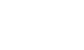(3marks)
2. Find the equation of a straight line that passes through the points A (2,-3) and B-5,1). Express your answer in the form ax + by = c where a, b and care integers. (3marks)
3. Solve for θ if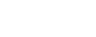(3marks)
4. A Swimming pool can be emptied by 3 pipes P, Q and working together in 33/4 hours. Pipe P and working alone takes 71/2 hours and 111/4 respectively. Determine how long pipe R working alone would take to empty the swimming pool. (3marks)
5. In the figure below PQ || RS.PS and RQ intersect at T. If PT: PS = 2:5 and QT = 3.5 cm, calculate correct to 2 decimal place RQ. (3 marks)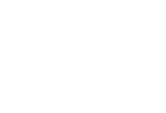6. A is the point (2, 3, 4) and B is the point (X, 6, 8).Determine the possible values of X if |AB| =13 (4 marks)
7. A metal hemisphere of radius 16 cm is melted down and cast into a cone of radius 8cm.Calculate the volume of the cone. (3marks)
8. Five of the iterior angles in a nonagon are 160° and the remaining are each x0. Find the possible values of x. (3marks)
9. Calculate the quartile deviation of the following set of data (4marks)
 Marks 21-30 31-40 41-50 51-60 61-70 71-80 81-90 91-100 No. of students 3 5 8 12 15 11 9 4
10. A metallic pipe which is 21 meters long has an internal radius of 13 cm and an external radius of 15 cm. if the density of the metal is 8000 kg/m3,  find its mass. (Take π = 22/7). (4 marks)
11. Muthoni, Chebet and Amina contributed ksh 50,000, ksh 40,000 and ksh 25,000 respectively to start a business. After some time they realized a profit which was shared in the ratio of their contribution. If Aminas share was ksh 10,000, by how much was Muthoni's share more than that of Chebet's. (3marks)
12. The cost of two jackets and 3 shirts was 1800. After the cost of a jacket and that of a shirt were increased by 20%, the cost of 6 jackets and 2 shirts was ksh 4,800. Calculate new total cost of 5 jackets and 4 shirts. (3 marks)
13. Without using mathematical tables evaluate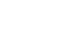(3marks)
14. Using a ruler and a pair of compass only, construct a rhombus AB< CD such that AC = 10 cm and ∠BAD = 60° (3marks)
15. Without using a calculator or mathematical tables, evaluate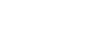(3marks)
16. A point P divides a line AB externally in the ratio 4:3. Given that A is (-2, 4) and point B (2,-3). Find the coordinates of T. (3marks)

SECTION II (50MARKS)
Answer only five questions from this section.

1. In the figure below, AC=14 cm, AD = 16 cm, DC = 11 cm, and B is a point on AC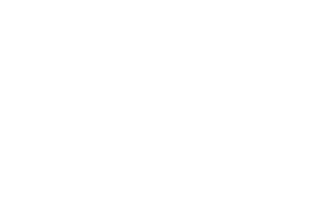1. Calculate, correct to 2d.p
2. The size of obtuse ∠ABD (3marks)
2. Calculate correct to 1 decimal place:
1. The length of AB (2marks)
2. The area of triangle BCD (2marks)
2. The following table shows heights of 100 seedlings each measured to the nearest cm.
 Height (cm) Frequency 70 - 79 14 80 - 84 16 85 - 89 18 90 - 94 20 95 - 99 17 100 - 109 15
1. Calculate the differences between the mean and the median. (6marks)
2. Draw a frequency polygon to illustrate the above information (4marks)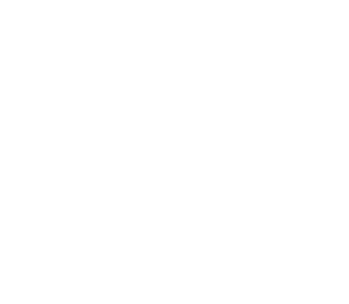3. Two towns P and Q are 280 km apart. A bus left town P at 9.30 am and travelled to Q at an average speed of 80 km/h. After 30 minutes, a car left town p for Q and travelled at an average speed of 100 km/h.
1. Determine:
1. The time when the car caught up with the bus. (3marks)
2. The distance of the car from town Q when it overtook the bus. (3marks)
2. After the car overtook the bus, it accelerated for 6 minutes to a speed of 120 km/h. It moved with that speed for 30 minutes after which breaks are applied and came to rest at town T after 3 minutes. Determine the distance travelled by the car in 39 minutes. (4marks)
4.
1. Complete the table of values for the equation y .-2x2 + 3x + 6. (2 marks)
 x -3 -2 -1 0 1 2 3 4 y
2. Use the values above to draw the graph of y = -2x2 + 3x + 6. (3marks)3. Using the graph drawn above Solve the oquations:
1. 2x2 = 3x +6 (2 marks)
2. -2x2 + x + 9 = 0 (3marks)
5. A trader deals with two types of Millet, type A and type B. type A costs ksh 400 per bag and type B costs ksh 350 per bag.
1. The trader mixes 30 bags of type A and 50 bags of type B. If she sells the mixture at a profit of 20%, calculate the selling price of one bag of the mixture. (4 marks)
2. The trader now mixes type A and type B in the ratio x: y respectively. If the cost of the mixture is ksh 383.50 per bag, find the ratio x:y. (4marks)
3. The trader mixes one bag of the mixture in part (a) with one bag of the mixture in part (b) above. Calculate the ratio of type A millet to type B millet in this mixture. (2marks)
6. The equation of a line L1 is 3y + 2x = 10
1. Find in form of y=mx+c,where m and care constants:
1. The equation of line L2 passing through N (-5,2) and parallel to L1 (2 marks)
2. The equation of line L3 perpendicular to L2 at M (1,-8) (3marks)
2. Find the angle of inclination of the line L, with the horizontal. (2marks)
3. Find the magnitude of MN. (3marks)
7. In the figure below A, B, C and D are points on the circle Centre O. ACF and EDFG are straight lines. Line EG is a tangent to the circle at D. ∠CDF = 35° and ∠CFG = 130°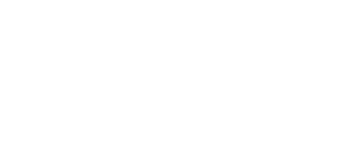1. Calculate the Size of
1. ∠OCD (2 marks)
2. ∠EDA (1 mark)
3. ∠ABC (2 marks)
2. Given that CFo 6.7 cm and DF = 8.5 cm, Calculate to 3 significant figures:
1. The length of DC. (3marks)
2. The radius of the circle. (3marks)
8. The product of the first three terms of a geometric progression is 64.If the first term is a and the common ratio is r,
1. Express r in terms of a. (3marks)
2. Given that the sum of the three terms is 14
1. Find the value of a and r and hence write down two possible sequence up to the 4th term. (5 marks)
2. Find the product of the 50th terms of the two sequence. (2marks)

### MARKING SCHEME

SECTION 1 (50 MARKS)
Answer all the questions in the space provided below each question

1. Without using mathematical tables or calculator evaluate(3marks)
11/3 - 7/3 x 5/6 x 3/2
11/3 - 35/12
44 - 35
12
9/12
3/4
Denominator
-1/8 - 1/4
- 1 - 2
8
-3/8
3/4 x 8/-3
= -2
2. Find the equation of a straight line that passes through the points A (2,-3) and B-5,1). Express your answer in the form ax + by = c where a, b and care integers. (3marks)
1 - 3 = -1
- 5 - 2    7
y + 3 = -4
x - 2    7
-4 (x - 2) = 7 (y + 3)
-4x + 8 = 7y + 21
-4x - 7y = 13
4x + 7y = -13
3. Solve for θ if(3marks)
sin(2θ - 50º) = cos (θ + 10º)
sin (2θ - 50º) = sin 90 - (θ + 10º)
20 -50 = 90 - θ - 10
3θ = 130
θ = 431/3 or 43.33º
4. A Swimming pool can be emptied by 3 pipes P, Q and working together in 33/4 hours. Pipe P and working alone takes 71/2 hours and 111/4 respectively. Determine how long pipe R working alone would take to empty the swimming pool. (3marks)
ans = 221/2 hrs
5. In the figure below PQ || RS.PS and RQ intersect at T. If PT: PS = 2:5 and QT = 3.5 cm, calculate correct to 2 decimal place RQ. (3 marks)RT/3.5 = 3/2
RT = 3/2 x 3.5
= 5.25
RQ = 5.25 + 3.5
= 8.75
6. A is the point (2, 3, 4) and B is the point (X, 6, 8).Determine the possible values of X if |AB| =13 (4 marks)
(x - 2)2 + (6 - 3)2 + (8 - 4)2 = 169
x2 - 4x + 4 + 9 + 16 = 169
x2 - 4x - 140 = 0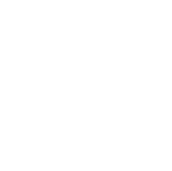7. A metal hemisphere of radius 16 cm is melted down and cast into a cone of radius 8cm.Calculate the volume of the cone. (3marks)
1/2 x 4/3 x 22/7 x 163 = 1/3 x 22/7 x 64 x h
h = 1/2 x 4/3 x 3 x 7 x 22/7 x 163
64 x 22
h = 128
volume of cone = 1/3 x 22/7 x 64 x 128
= 8,582
8. Five of the interior angles in a nonagon are 160° and the remaining are each x0. Find the possible values of x. (3marks)
sum of interior angles of  nonagon = 180(9 - 2)
= 1260
160 x 5 + 4x = 1260
4x = 1260 - 800
x = 460
4
x = 115
9. Calculate the quartile deviation of the following set of data (4marks)
 Marks 21-30 31-40 41-50 51-60 61-70 71-80 81-90 91-100 No. of students 3 5 8 12 15 11 9 4 cf 3 8 16 28 43 54 63 67
Q1 = 1/4 x 67 = 16.75
50.5 + (16.75 - 16)10
12
50.5 + 0.625
∠ 1.125
Q3 = 3/4 x 67 = 50.25
70.5 + (50.25 - 43) x 10
11
70.5 + 6.591
= 77.09
Quartile deviation =
77.09 - 51.125 = 25.965
2                     2
= 12.98
10. A metallic pipe which is 21 meters long has an internal radius of 13 cm and an external radius of 15 cm. if the density of the metal is 8000 kg/m3,  find its mass. (Take π = 22/7). (4 marks)
external volume = 22/7 x 7.52 x 2100 = 371,250
internal volume = 22/7 x 6.52 x 2100 = 278,850
volume of material = 371,250 - 278,250 = 92,400cm3
= 0.924m3
8000 = mass
0.924
mass = 7,392 kg
11. Muthoni, Chebet and Amina contributed ksh 50,000, ksh 40,000 and ksh 25,000 respectively to start a business. After some time they realized a profit which was shared in the ratio of their contribution. If Aminas share was ksh 10,000, by how much was Muthoni's share more than that of Chebet's. (3marks)
M:C:A = 50000:40000:25000
=10:8:5
5/23 x 10000
x = 10000 x 23
5
= 46000
muthoni  = 10/23 x 46000 = 20000
chebet = 8/23 x 46000 = 8000
20000- 8000
= 12000
12. The cost of two jackets and 3 shirts was 1800. After the cost of a jacket and that of a shirt were increased by 20%, the cost of 6 jackets and 2 shirts was ksh 4,800. Calculate new total cost of 5 jackets and 4 shirts. (3 marks)
(2J + 3S = 1800)8
(7.2J + 2.4S = 4800)10
16J + 24S = 14400
72J + 24S = 48000
56J                    =  33600
= 600
shrt = 1800 - 1600 = 200
3
1.2 x 600 = 720
1.2 x 200 = 240
5 x 720 + 4 x 240
3600 + 960
= 4560
13. Without using mathematical tables evaluate(3marks)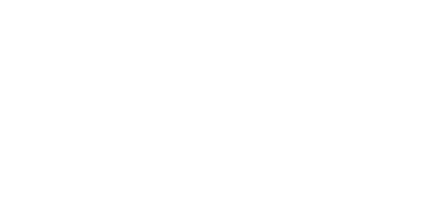14. Using a ruler and a pair of compass only, construct a rhombus ABCD such that AC = 10 cm and ∠BAD = 60° (3marks)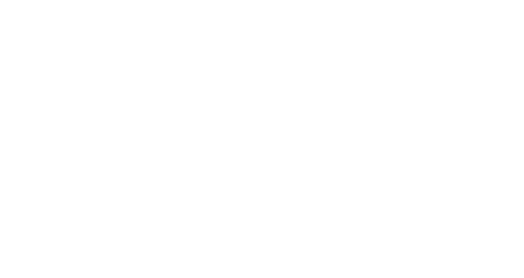15. Without using a calculator or mathematical tables, evaluate(3marks)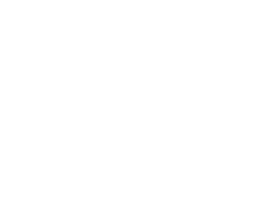16. A point P divides a line AB externally in the ratio 4:3. Given that A is (-2, 4) and point B (2,-3). Find the coordinates of T. (3marks)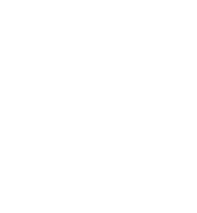T(14, -24)

SECTION II (50MARKS)
Answer only five questions from this section.

1. In the figure below, AC=14 cm, AD = 16 cm, DC = 11 cm, and B is a point on AC1. Calculate, correct to 2d.p
112 = 142+ 162 - 2 x 14 x 16 x cosA
121 = 196 + 256 - 448 cos A
448 cos A = 331
cos A = 0.7388
A = 42.37
1. The size of obtuse ∠ABD (3marks)
16/sin B = 12/sin 42.37
sin B = 0.8986
B = 63.97

obtuse angle B = 116.03
2. Calculate correct to 1 decimal place:
1. The length of AB (2marks)
ADB = 180 - (42.37 + 116.03)
= 21.6º
AB          =            16
sin 21.6            sin 116.03
AB = 6.555
AB = 6.6
2. The area of triangle BCD (2marks)
DBC = 180 - 116.03 = 63.97, BC = 14 - 6.555
= 7.445
1/2 x 7.445 x 12 x sin 63.97
40.14
40.1
2. The following table shows heights of 100 seedlings each measured to the nearest cm.
 Height (cm) Frequency x fx cf frequency class 70 - 79 14 74.5 1043 14 1.4 80 - 84 16 82 1312 30 3.2 85 - 89 18 87 1566 48 3.6 90 - 94 20 92 1840 68 4.0 95 - 99 17 97 1649 85 3.4 100 - 109 15 104.5 1567.5 100 1.5 Σf = 100 Σfx = 89775
1. Calculate the differences between the mean and the median. (6marks)
x = 8977.5 = 89.78
100
median = 89.5 + (50 - 48) x 5
20
= 89.5 + 0.5
= 90
differences = 90 - 89.78
= 0.22
2. Draw a frequency polygon to illustrate the above information (4marks)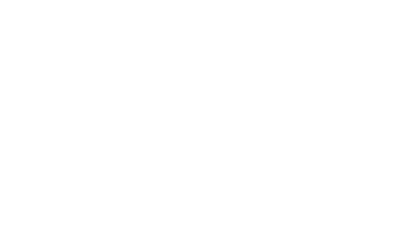3. Two towns P and Q are 280 km apart. A bus left town P at 9.30 am and travelled to Q at an average speed of 80 km/h. After 30 minutes, a car left town p for Q and travelled at an average speed of 100 km/h.
1. Determine:
1. The time when the car caught up with the bus. (3marks)
distance moved by bus
30/60 x 80 = 40 km
R.s = 100 - 80 = 20km/h
Time = 40/20 = 2hrs
9:30
2:00
11:30 am
2. The distance of the car from town Q when it overtook the bus. (3marks)
distance = 2 x 100 = 200 km
dixtance frm Q = 280 - 200
= 80 km
2. After the car overtook the bus, it accelerated for 6 minutes to a speed of 120 km/h. It moved with that speed for 30 minutes after which breaks are applied and came to rest at town T after 3 minutes. Determine the distance travelled by the car in 39 minutes. (4marks)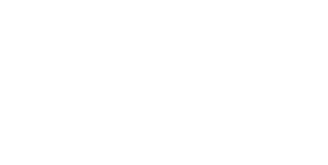1/2 x 6/60(100 + 120) + 30/60 x 120 + 1/2 x 3/60 x 120
11 km + 60 km + 3 km
= 74 km
4.
1. Complete the table of values for the equation y .-2x2 + 3x + 6. (2 marks)
 x -3 -2 -1 0 1 2 3 4 y -21 -8 1 6 7 4 -3 -14
2. Use the values above to draw the graph of y = -2x2 + 3x + 6. (3marks)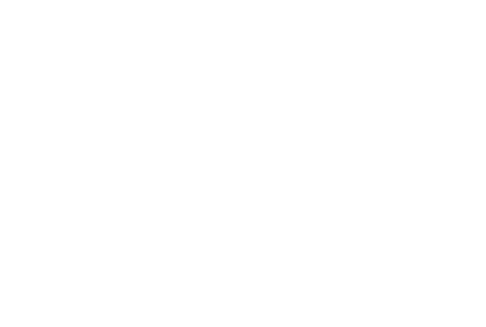3. Using the graph drawn above Solve the oquations:
1. 2x2 = 3x +6 (2 marks)
-2x2 + 3x + 6 = 0
at point of intersection of curve with x-axis
x = -1.15  or x = 2.1
2. -2x2 + x + 9 = 0 (3marks)
x = -1.85 or 2.35
5. A trader deals with two types of Millet, type A and type B. type A costs ksh 400 per bag and type B costs ksh 350 per bag.
1. The trader mixes 30 bags of type A and 50 bags of type B. If she sells the mixture at a profit of 20%, calculate the selling price of one bag of the mixture. (4 marks)
30 x 400 + 50 x 350
80
29500
80
368.75
100% - 368.75
120% - 120 x 368.75
100
= 442.50
2. The trader now mixes type A and type B in the ratio x: y respectively. If the cost of the mixture is ksh 383.50 per bag, find the ratio x:y. (4marks)
400x + 350y = 383.50
x + y
400x + 350y = 383.50x + 383.50y
16.5x = 33.5y
x/y = 33.5/16.5
x/y = 21/33
x:y = 67:33
3. The trader mixes one bag of the mixture in part (a) with one bag of the mixture in part (b) above. Calculate the ratio of type A millet to type B millet in this mixture. (2marks)
A:B = 3:5        A:B = 67:33
A 3/8                 A 67/100
B 5/8                B 33/100
3/8 + 67/100 : 5/8 + 33/100
19/200 : 191/200
209/200: 191/200
209:191
6. The equation of a line L1 is 3y + 2x = 10
1. Find in form of y=mx+c,where m and care constants:
1. The equation of line L2 passing through N (-5,2) and parallel to L1 (2 marks)
3y = -2x + 10
y = -2x/3 + 10/3
Q1 = -2/3 = a2
y - 2 = -2
x + 5    3
-2x - 10 = 3y - 6
-2x - 4 = 3y
y = -2x/3 - 4/3
2. The equation of line L3 perpendicular to L2 at M (1,-8) (3marks)
y = 3/2x - 19/2
2. Find the angle of inclination of the line L, with the horizontal. (2marks)
tan θ = -2/3
θ = 33.69
but tan is -ve
therefore θ = 146.3
3. Find the magnitude of MN. (3marks)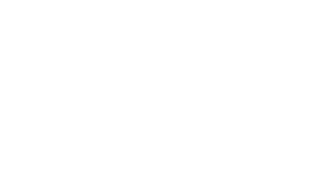7. In the figure below A, B, C and D are points on the circle Centre O. ACF and EDFG are straight lines. Line EG is a tangent to the circle at D. ∠CDF = 35° and ∠CFG = 130°1. Calculate the Size of
1. ∠OCD (2 marks)
1/2(180 - 70) = 55º
2. ∠EDA (1 mark)
ACD = EDA = 30 + 55 = 85º
3. ∠ABC (2 marks)
∠ ADC = 55 + 5 = 60º
∠ABC = 180 - 60 = 120º
2. Given that CFo 6.7 cm and DF = 8.5 cm, Calculate to 3 significant figures:
1. The length of DC. (3marks)
DC2 = 8.52 + 6.72 - 2 x 6.7 x 8.5 x cos 50
= √43.93
= 6.628
= 6.63
2. The radius of the circle. (3marks)
r = 5.78
8. The product of the first three terms of a geometric progression is 64.If the first term is a and the common ratio is r,
1. Express r in terms of a. (3marks)
2. Given that the sum of the three terms is 14
1. Find the value of a and r and hence write down two possible sequence up to the 4th term. (5 marks)
2. Find the product of the 50th terms of the two sequence. (2marks)

• ✔ To read offline at any time.
• ✔ To Print at your convenience
• ✔ Share Easily with Friends / Students